# Delete Every Other Row

This post will guide you how to delete every other row by filter command in Excel. How do I delete every other row in a selected range on a worksheet by Excel VBA Macro.

Delete Every Other Row with Filter command

To delete every other row in Excel, you need to filter alternate rows, and then select those rows, and delete them at once. So you need to create a helper column using MOD function with ROW function, just do the following steps:

#1 type the formula =MOD(ROW(),2) in one cell next to your original data, and press enter key, then drag the auto fill handler over other cells to generate 0,1 numbers in each cells.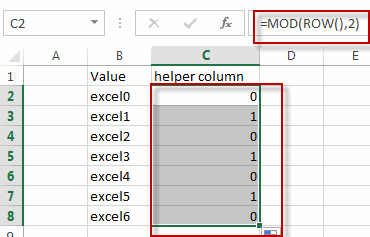#2 select the helper column, go to DATA tab, Click Filter command under Sort & Filter group. The drop-down filter arrows will appear in header cells.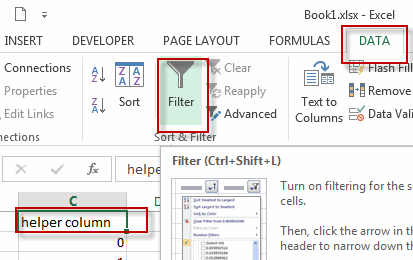#3 click the filter arrow in the helper column, and select the 0 check box from the filter drop down list.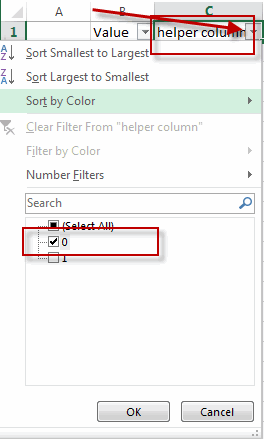#4 you will see that all “1” rows are hidden, select all the visible “0” rows, then right-click on the selection range, and click Delete Row.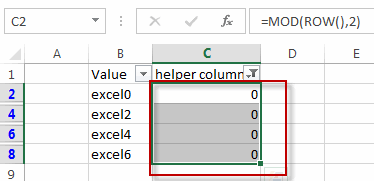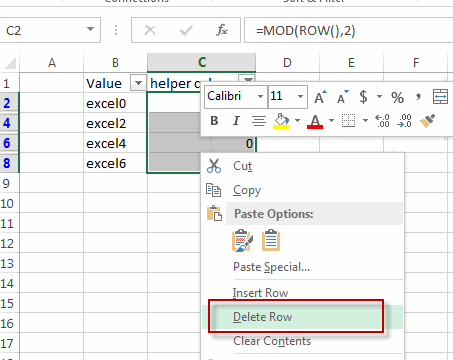#5 all rows with “0” values are removed. Then click the Filter button again on the helper column to remove auto-filter.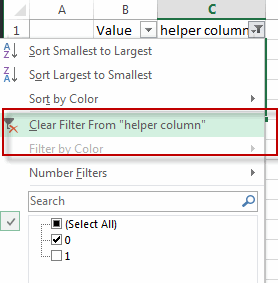#6 you can delete the helper column.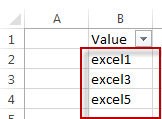Delete every other row with VBA

You can also use an Excel VBA Macro to delete every other row. Do the following steps:

#1 click on “Visual Basic” command under DEVELOPER Tab.#2 then the “Visual Basic Editor” window will appear.

#3 click “Insert” ->”Module” to create a new module.#4 paste the below VBA code into the code window. Then clicking “Save” button.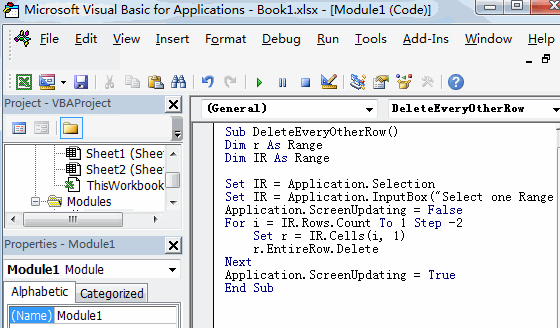```Sub DeleteEveryOtherRow()
Dim r As Range
Dim IR As Range

Set IR = Application.Selection
Set IR = Application.InputBox("Select one Range :", "Delete Every Other Row", IR.Address, Type:=8)
Application.ScreenUpdating = False
For i = IR.Rows.Count To 1 Step -2
Set r = IR.Cells(i, 1)
r.EntireRow.Delete
Next
Application.ScreenUpdating = True
End Sub```

#5 back to the current worksheet, then run the above excel macro. Click Run button.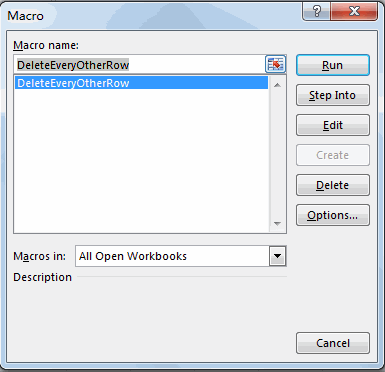#6 Select one range that you want to delete every other row.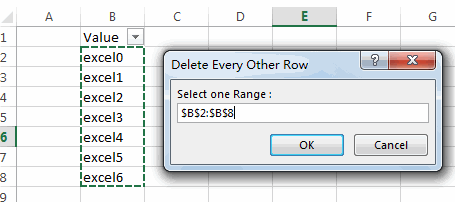#7 let’s see the result.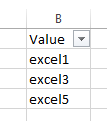### Related Functions

• Excel ROW function
The Excel ROW function returns the row number of a cell reference.The ROW function is a build-in function in Microsoft Excel and it is categorized as a Lookup and Reference Function.The syntax of the ROW function is as below:= ROW ([reference])….
• Excel MOD function
he Excel MOD function returns the remainder of two numbers after division. So you can use the MOD function to get the remainder after a number is divided by a divisor in Excel. The syntax of the MOD function is as below:=MOD (number, divisor)….